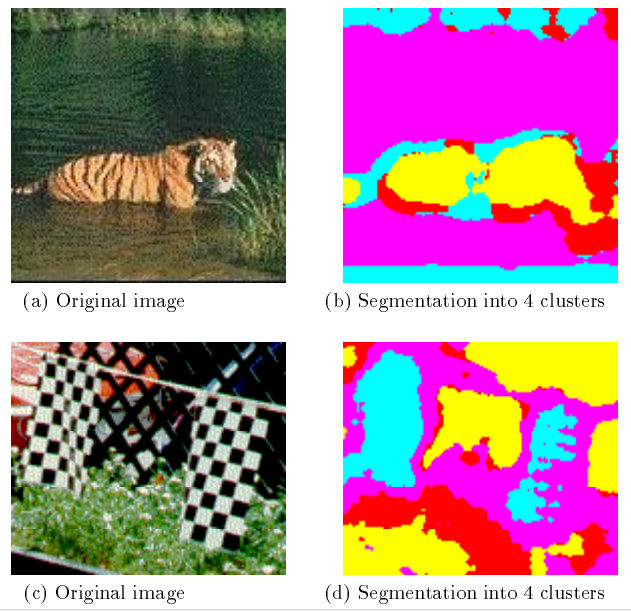# TensorFlow 101C. Image Texture

This Note is for image texture explanation:

1. Reference

https://courses.cs.washington.edu/courses/cse576/book/ch7.pdf  (computer vision)

1. Why Texture

Texture gives us information about the spatial arrangement of the colors or intensities in an image. Why?

The answer is the histogram can’t fully represent/classify images. All images below are half white and half black. However, the images are different.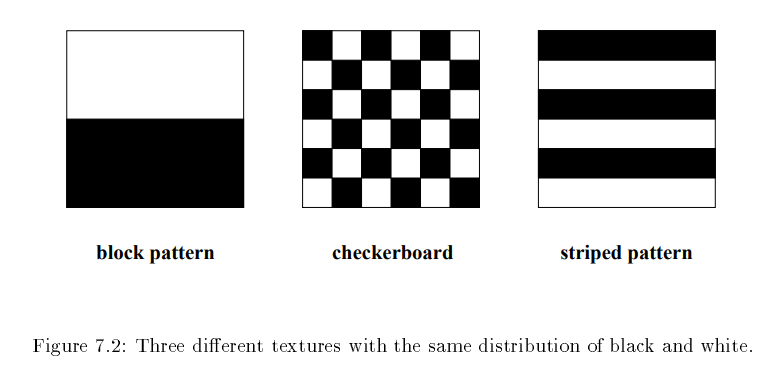1. How to recognize texture
1. Structural approach: Texture is a set of primitive texels in some regular or repeated relationship.
2. Statistical approach: Texture is a quantitative measure of the arrangement of intensities in a region.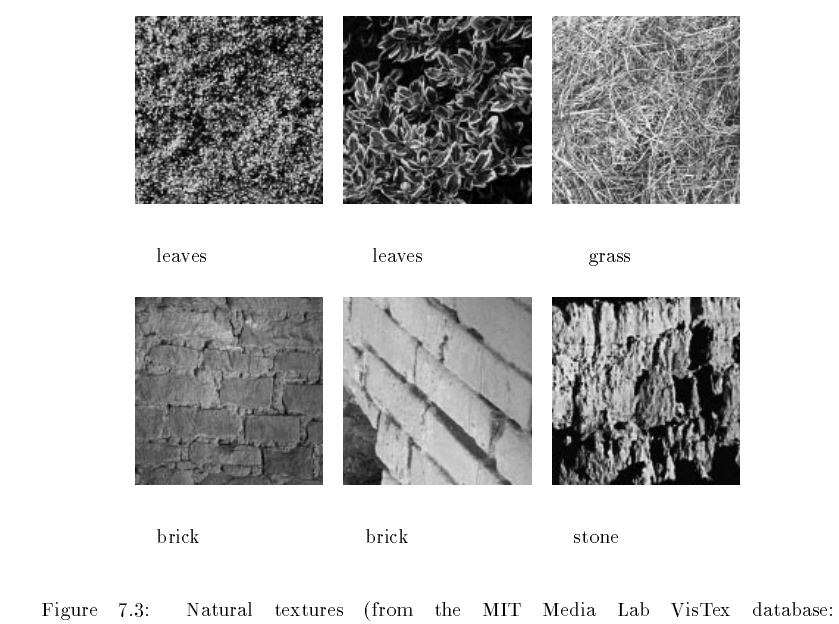1. Statistical method
1. Co-occurrence Matrices and Feature
1. Basic concept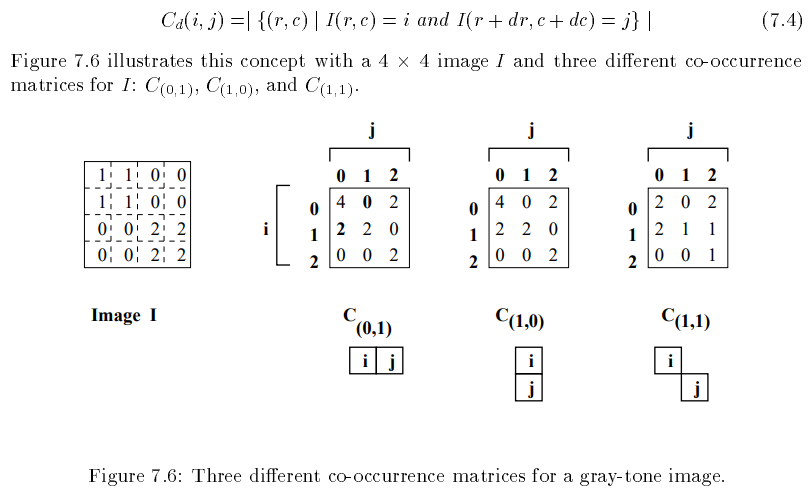1. Explanation in data:

In the matrix of C(0,1), the first row is 4, 0, 2.  (Which measure the occurrence of the values of two adjacent cells in the same row)

[0,0]=4 means: In the two adjacent cells (in the same row) of Image I, the # of of occurrence of (the left cell =0 and the right cell=0) is 4, as shown the red rectangles in image below.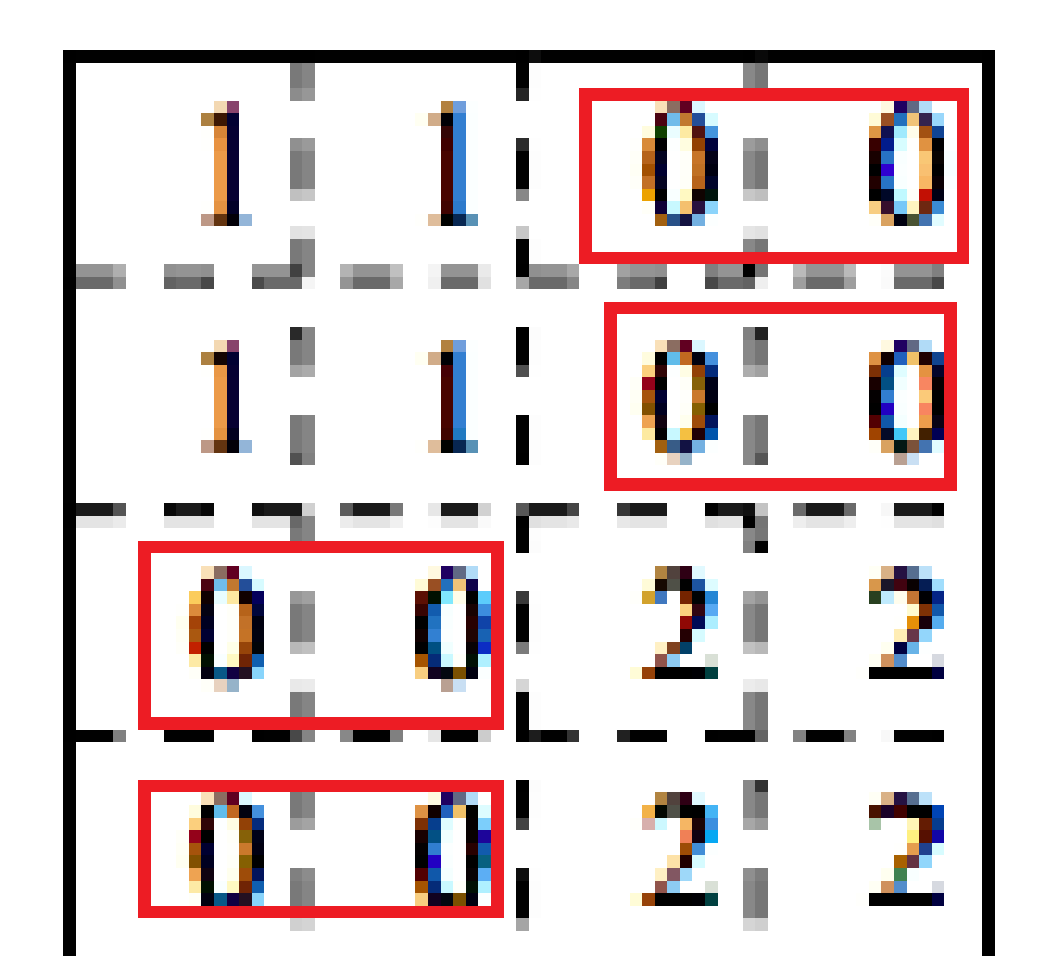[0,1]=0 means: in the two adjacent cells (in the same row) of Image I, the # of of occurrence of (the left cell =0 and the right cell=1) is 0.

[0,2]=2 means: in the two adjacent cells (in the same row) of Image I, the # of of occurrence of (the left cell =0 and the right cell=2) is 2.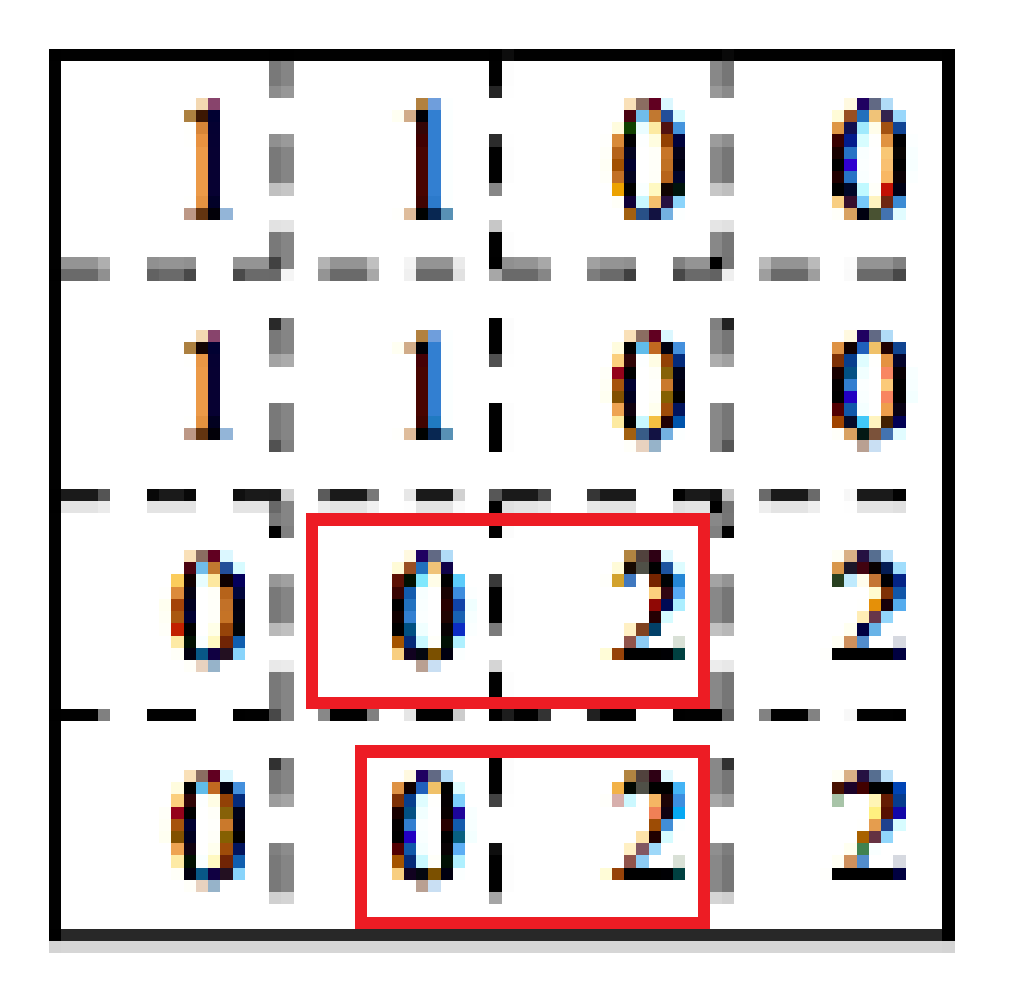1.  Probability of the occurrence: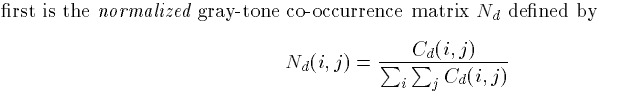1.  Co-occurrence matrices capture properties of a texture, but they are not directly useful

For further analysis, such as comparing two textures. Instead, numeric features are computed from the co-occurrence matrix that can be used to represent the texture more compactly. The following are standard features derivable from a normalized co-occurrence matrix.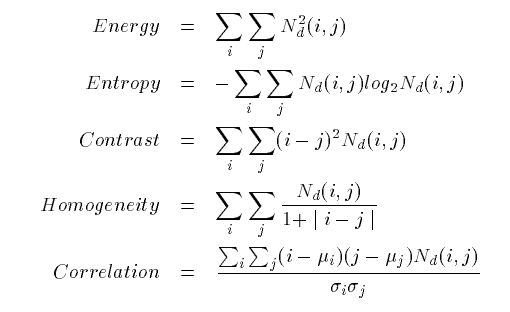1.  Laws’ Texture Energy Measure

Another approach to generating texture features is to use local masks to detect various Types of texture. Laws developed a texture-energy approach that measures the amount of variation within a fixed-size window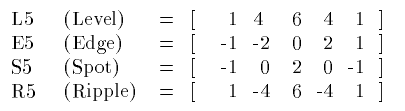Figure 7.8 shows the segmentation of several images using

The Laws texture energy measures and a clustering algorithm to group pixels into regions